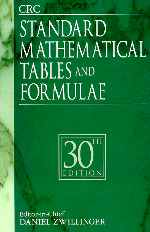SMTFSMTF

 What color is the cover of your copy of Mathematical Tables and Formulae? Images of previous covers are here.33nd print edition
• 33rd Edition, D. Zwillinger (ed.), Chapman and Hall/CRC, Boca Raton, 2018, 872 pages, ISBN 9781498777803
• New short sections include:
• Bar Codes
• Braid groups
• Card Games
• Compressive Sensing
• Constrained Least Squares
• Continuous Wavelet Transform
• Contour integration
• Coupled Analogues
• Divisibility tests
• Electromagnetic Field Equations
• Financial options
• Formulas from the sciences: Biological models, Chemistry, Radar
• Fractal Arithmetic
• Functions: Lambert, prolate spheroidal, Weierstrass elliptic
• General Relativity
• Generating functions
• Linear Fractional Transformations
• Linear Temporal Logic
• Matrix Pseudospectra
• Max Plus Algebra
• Numerals in Different Languages
• Ones and Twos Complement
• Proof Methods
• Random Matrices
• Separation of Variables for Klein-Gordon equation
• Significant Mathematical Equations
• Current errata in PDF format32nd print edition
• 32nd Edition, D. Zwillinger (ed.), CRC, Boca Raton, 2011, 833 pages, ISBN 1-439835489
• There are new short sections on:  Bezier curves Euclidean geometry cellular automata cryptography elliptic curves knot theory lattices music p-adic numbers polyominoes rational trigonometry rook polynomials
• New statistics tables included: circular normal probability, contingency tables, estimators sample sizes, runs test, sample size
• There is a new chapter, "Mathematical Formulae from the Sciences", with short sections on:  Acoustics Astrophysics Atmospheric Physics Basic Mechanics Beam dynamics Classical mechanics Coordinate systems Earthquake Engineering Electronic circuits E & M Epidemiology Finance Fluid mechanics Fuzzy logic Human body Image Processing Matrices Macroeconomics Physical Systems Optics Population Genetics Quantum mechanics Quaternions Relativistic Mechanics Solid Mechanics Statistical mechanics Thermodynamics
• Current errata in PDF format31st print edition
• 31st Edition, D. Zwillinger (ed.), CRC, Boca Raton, 2003, 910 pages, ISBN 1-58488-291-3
• Improvements included:
• Tables of cycle index and colorings from Polya theory
• Table of cyclotomic polynomials
• Table of factorization of xn-1 over F2
• Table of Zernike polynomials
• Group tables for small groups
• Details on the Jacobian elliptic functions
• Current errata in PDF format
• An addendum (in pdf) may be viewed here; it contains:
• tables of voting power for small games
• the cyclic ruleElectronic edition
• Created from 30th print edition30th print edition
• 30th Edition, D. Zwillinger (ed.), CRC, Boca Raton, 1995, 821 pages, ISBN 0-8493-2479-3
• Chinese version of 30th edition created in 1998.29th print edition